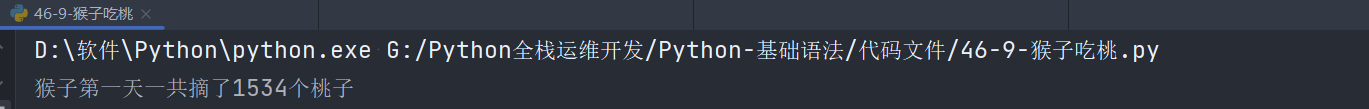##MSIPO技术圈 首页 IT技术 查看内容

# 第63讲：Python编程案例之猴子吃桃

2023-07-13

## 1.需求描述以及分析

• 第一天吃掉了一半的桃子，还剩下一半，又多吃了一个，第二天吃了第一天所剩桃子数的一半，并且也多吃了一个。
• 那么就得到：第1天的桃子个数是第2天所吃的桃子个数加1后的2倍，第2天的桃子个数是第3天所吃的桃子个数加1后的2倍，依次类推，直到只剩下1个桃子。第n天的桃子数就是第n+1天所吃的桃子个数加1后的2倍。

## 2.递推方式实该该程序

• 已知第1天的桃子个数是第2天所吃的桃子个数加1后的2倍，第2天的桃子个数是第3天所吃的桃子个数加1后的2倍。

• 我们可以将每天的桃子数放在一个列表`mp`里，在列表中填充11个None值，只有10天，填充11个元素的目的是，索引为0的元素不会被处理，因此要填充11个元素，已知第10天的桃子数为1，那么就将索引为10的元素，初始化成1：`mp=1`

• 可以设`n`天的桃子数为`mp[n]`，递推表达式就是：`mp[n]=(mp[n+1]+1)*2`，且`mp=1`

• 确定好表达式之后，我们就可以写一个for循环，已知第10天为1个桃子了，那么就从第9天开始循环，已知递推循环到第1天，每循环一次，就根据递推表达式，计算出这天的桃子树，递推表达式会将后一天的桃子数加1并且乘以2，因此不用手动累加每天的桃子个数，到了第一天时，一定是准确的桃子个数。

例如第一次循环时第9天，根据递推表达式`mp = (mp[9+1] + 1) *2` = `mp = (mp + 1) = 2 * 2 = 4 `，第9天的桃子数就是4个，以此类推。

``````def monkey_peach():
#首先定义一个列表，存放11个元素，表示猴子每天的桃子数量，放11个是因为索引为0的元素不会被处理，10天，一直要处理到索引为10的元素，因此要放11个元素
mp = [None] * 11
#已知第10天的桃子数量为1，因此直接给第10天赋值为1，表示1个桃子
mp = 1
#第10天不用处理，从第9天开始循环遍历，一直到第1天，需要采用逆推的方式，因此步长为-1
for n in range(9, 0, -1):
#带着每次循环的天数，去套逆推表达式，得到本天的桃子数量
mp[n] = (mp[n + 1] + 1) * 2
#由于当天的桃子数量都是后一天桃子数+1的2倍，因此无需对列表中的元素进行累加，求第一天摘了多少个桃子，直接返回索引为1的元素即可
return mp

print('猴子第一天一共摘了{}个桃子'.format(monkey_peach()))
``````## 3.递归方式实现该程序

``````def monkey_peach(day):
if day == 10:
return 1
else:
return (monkey_peach(day + 1) + 1) * 2

print('猴子第一天一共摘了{}个桃子'.format(monkey_peach(1)))
``````1）首先开始递推

• 调用函数时传入day的实参为1，即day=1，视作为day1。
• 开始第一次递推：day+1=1+1=2，即day1+1，视作为day2。
• 开始第二次递推：day+1=2+1=3，即day2+1，视作为day3。
• 开始第三次递推：day+1=3+1=4，即day3+1，视作为day4。
• 开始第四次递推：day+1=4+1=5，即day4+1，视作为day5。
• 开始第五次递推：day+1=5+1=6，即day5+1，视作为day6。
• 开始第六次递推：day+1=6+1=7，即day6+1，视作为day7。
• 开始第七次递推：day+1=7+1=8，即day7+1，视作为day8。
• 开始第八次递推：day+1=8+1=9，即day8+1，视作为day9。
• 开始第九次递推：day+1=9+1=10，10满足递归结束条件：`if day == 10`，此时递推结束，第九次递推拿到return返回值1。

2）然后带着返回值开始回归遍历计算

• 带着第九次递推的返回值去回归。

• 回归到第八次递推，即day9，此时day9的结果就是`((day9+1)+1)*2`，day9+1为day10，day10的值1，然后套入公式中，即`day9=(1+1)*2=4`

• 回归到第七次递推，即day8，此时day8的结果就是`((day8+1)+1)*2`，day8+1为day9，day9的值为4，然后套入公式中，即`day8=(4+1)*2=10`

• 回归到第六次递归，即day7，此时day7的结果就是`((day7+1)+1)*2`，day7+1为day8，day8的值为10，然后套入公式中，即`day7=(10+1)*2=22`

• 回归到第五次递推，即day6，此时day6的结果就是`((day6+1)+1)*2`，day6+1为day7，day7的值22，然后套入公式中，即`day6=(22+1)*2=46`

• 回归到第四次递推，即day5，此时day5的结果就是`((day5+1)+1)*2`，day5+1为day6，day6的值为46，然后套入公式中，即`day5=(46+1)*2=94`

• 回归到第三次递归，即day4，此时day4的结果就是`((day4+1)+1)*2`，day4+1为day5，day5的值为94，然后套入公式中，即`day4=(94+1)*2=190`

• 回归到第二次递推，即day3，此时day3的结果就是`((day3+1)+1)*2`，day3+1为day4，day4的值190，然后套入公式中，即`day3=(190+1)*2=382`

• 回归到第一次递推，即day2，此时day2的结果就是`((day2+1)+1)*2`，day2+1为day3，day3的值为382，然后套入公式中，即`day2=(382+1)*2=766`

• 到此递推和回归就结束了，带着返回值766与调用函数传递的day1进行运算，day1的结果就是`((day1+1)+1)*2`，day1+1位day2，也就是递归函数的运算结果766，套入公式中即`day1=(766+1)*2=1534`

1534就是函数的返回值。